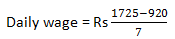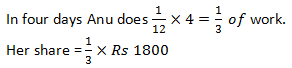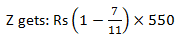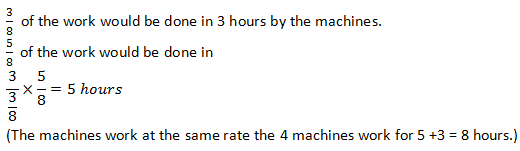# Time, Work and Wages Problems with Explanation

Time and Work is an extension of Time and Work chapter. In this chapter we convert work performed in monetary terms.I have already shared Time and Work tricks here.

THEOREM: Wages are given in proportion to the work done and in indirect (or inverse) proportion to the time taken by the individual.

### Question 1.

Ravi can do a work in 6 days and Rahim can do the same work in 5 days.The contract for the work is Rs. 220. How much shall B get if both of them work together?### Question 2.

A man can do a work in 10 days. With the help of a boy he can do the same work in 6 days. If they get Rs. 50 for the work, what is the share of the boy?### Question 3.

A, B and C can do a work in 6, 8 and 12 days respectively. Doing that work together they get an amount of Rs 1350. What is the share of B in that amount?### Question 4.

A, B and C contract a work for Rs. 550. Together, A and B are supposed to do 7/11 of the work. How much does C get ?### Question 5.

Two men undertake to do a piece of work for Rs. 200. One alone could do it in 6 days, the other in 8 days. With the assistance of a boy they finish it in 3 days. How should the money be divided?### Question 6.

A and B undertook to do a piece of work for RS 4500. A alone could do the work in the 8 days and B in 12 Days. With the assistance of C, they finished the work in 4 days. How should the money be divided?
Sol:### Question 7.

12 Men and 6 women can do a piece of work in 14 days, while 8 men and 9 women can do the work in 16 days. if a man gets Rs 54 per days, what should be the wage of a woman per day?
Sol:### Question 8.

If the wage of 45 women amount to Rs 15525 in 48 days, how many men must work for 16 days to receive Rs 5750, The daily wage of a man being double of that of a woman?
Sol:### Question 9.

A, B and C are employed to do a piece of work for Rs 529. A and B together are supposed to doof the work and rest is to be complete by C. What is the amount paid to C?
Sol:### Question 10.

A can do a piece of work in 10 days while B alone can do it in 15 days. They work together for 5 days and the rest of the work is done by C in 2 days. If they get Rs 450 for the whole work, how should they divide the money?
(a) Rs 100. Rs 150, Rs 200
(b) Rs 80, Rs 170, Rs 200
(c) Rs 225, Rs 150, Rs 75
(d) Rs 200, Rs 50, Rs 200
Ans: B Rs 225, Rs 150, Rs 75
Hint:### Question 11.

I engaged a man for a certain number of days for Rs 1725. He was absent for 7 days. I paid him Rs 920. What was his daily wage?
(a) Rs 315
(b) Rs 275
(c) Rs 215
(d) Rs 115
Ans: Rs 115.### Question 12.

Anu and her friend Radha undertook a piece of work for Rs 1800. Ann alone could do the work in 12 days and Radha in 18 days. With the assistance of Rama, they completed the work in 4 days. Find the share of Anu in the money, if the money is to be shared in proportion to the amount of work done.
(a) Rs 550
(b) Rs 600
(c) RS 650
(d) Rs 800
Ans: Rs 600### Question 13.

A and B undertook a job for Rs 1400. A can do it alone in 7 days and B in 8 days. With the help of C, they finish the work in 3 days. How should the money be divided?
(a) RS 600. RS 525. RS 275
(b) Rs 550, Rs 500, Rs 350
(c) Rs 630, Rs 490, Rs 280
(d) None of these
Ans:  Rs 600. Rs 525. Rs 275

### Question 14.

A, B and C completed a piece of work. A worked for 6 days. B for 9 days and C for 4 days. Their daily wages were in ratio of 3 : 4 : 5. Find the daily wage of C, if their total earning was Rs 1480.
(a) Rs 80
(b) Rs 75
(c) Rs 100
(d) Rs 90
Ans: Rs 600
The total money would be shared in the ratio 3 x 6 : 4 x 9: 5 x 4

### Question 15.

X, Y and Z contract a piece of work for Rs 550. X and Y together are to doof the work. How much would Z get for the work?
(a) RS 200
(b) Rs 250
(c)Rs 180
(d) Rs 160
Ans: Rs 200### Question 16.

X alone can finish a piece of work in 10 days while Y alone can do it in 15 days. If they undertook the job for Rs 225, the share of Y would be
(a) Rs 90
(b) Rs 100
(c) Rs 80
(d) Rs 75
Ans:(A) Rs 90

### Question 17.

X, Y and Z together do a piece of work for Rs 53.50. X working alone could do it in 5 days, Y working alone could do it in 6 days and Z working alone could do it in 7 days. How should the money be divided among them ?
(a) Rs 21. RS 17.50. Rs 15
(b) Rs 20, Rs 15.50, Rs 18
(c) Rs 25, Rs 10 .,50 Rs 18
(d) Rs 18, Rs 16.50, Rs 19
Ans:(A) Rs 21. RS 17.50. Rs 15

### Question 18.

In a company 4 machines are running simultaneously at a constant rate ‘r’ to produce ofthe total order for wrenches. The remaining wrenches needed as per the order are to be produced in 3 hours with all 4 machines running at the same rate ‘r’. If the cost of operating each machine for one hour is Rs 22.00, find the total cost of machine operation to meet the order.

(a) Rs 840
(b) Rs 704
(c) Rs 810
(d) Data insufficient
Ans: Rs 704### Question 19.

A sum of Rs 77 was paid for a piece of work which A can do in 10 days, B in 15 days, C in 20 days and D in 25 days. Find the share of C, if all of them worked together.
(a) Rs 12
(b) Rs 17
(c) Rs 15
(d) Rs 18
Ans: (C) Rs 15### Question 20.

3 men can do as much work as 5 boys. The wages of 3 boys are equal to those of 2 men. A piece of work for which 40 boys and 15 men are employed takes 8 weeks and costs Rs 15750. How long would it take if 20 boys and 20 men were employed and how much would it cost?  (a) 55 days, Rs 21600
(b) 68¼ days, Rs 15356.25
(c) 68¼ days, Rs 13800
(d) 50 days, Rs 13005.75
Ans: (B) 68¼ days, Rs 15356.25

### Question 21.

Wages for 45 women amount to Rs 15525 in 48 days. How many men must work 16 days to receive Rs 5750, the daily wages of a man being double those of a woman?Read Time and Work Shortcuts here#### What's trending in BankExamsToday

Smart Prep Kit for Banking Exams by Ramandeep Singh - Download here